# Standard Algorithm

Page 3 of 5

Ready to do one with a bigger number and more DMS loops?  I think you are, dude!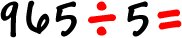Set it up: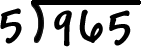Remember, your DMS loop...  Division, then multiplication, then subtraction...  and we do this until we run out of numbers!

Division:

 Five doesn't go into 9 cleanly... What comes close, but is a little less? Five goes into 9 one time... with a little left over.  Put that 1 right above the 9:That little freaky thing seemed a lot easier this time, didn't it?  Let's keep going!

 Multiplication: Multiply the 1 and the 5 and put the answer right under the 9: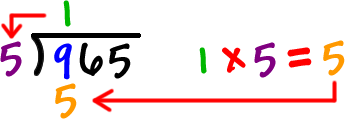Subtraction: Do the subtraction...That's 9 - 5 = 4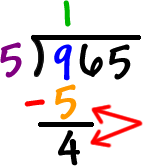First DMS loop done!  This is getting easier, isn't it?

Division, multiplication, subtraction.

Let's get the next number and do the DMS loop again!

 Set it up:  Drag the 6 down. That makes it a 46...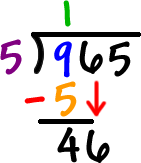Now, let's go back into our DMS loop using the 46!

 Division: We want to see how many times 5 will go into 46...  Five goes into 45 nine times, so we'll use that! 5 goes into 46 nine times with a little left over.  Put that 9 right above the 6: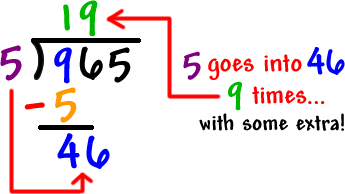Multiplication: Multiply the 9 and the 5 and put the answer right under the 46: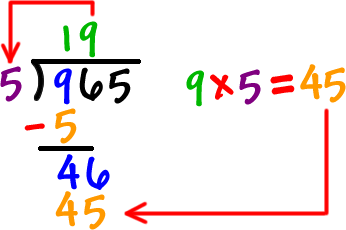Subtraction: That's 46 - 45 = 1 We haven't used all the numbers yet, so we need to do another loop!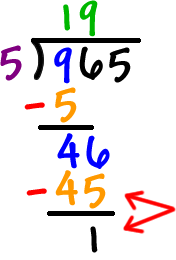Let's get the next number and do the DMS loop again!

 Get that bad boy set up:  Drag the 5 down. That makes it a 15...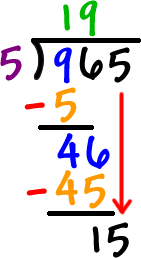Now, let's go back into our DMS loop using the 15!

 Division: We want to see how many times 5 will go into 15...  It goes in cleanly! 5 goes into 15 three times.  Put that 3 right above the 5: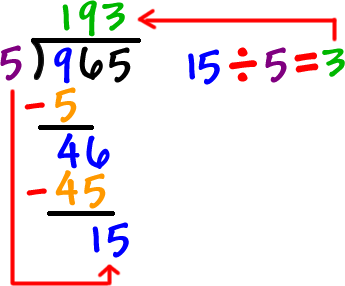Multiplication: Multiply the 3 and the 5 and put the answer right under the 15: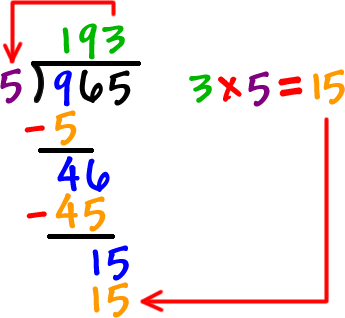Subtraction: That's 15 - 15 = 0 DONE, BABY!! The answer is 193.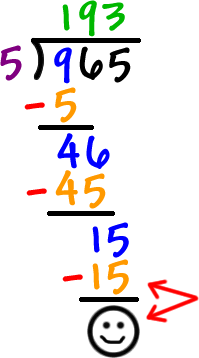Check it!!  193 x 5 should = 965!

There's one more little thing I need to show you before you try some on your own...  Check out the next page!